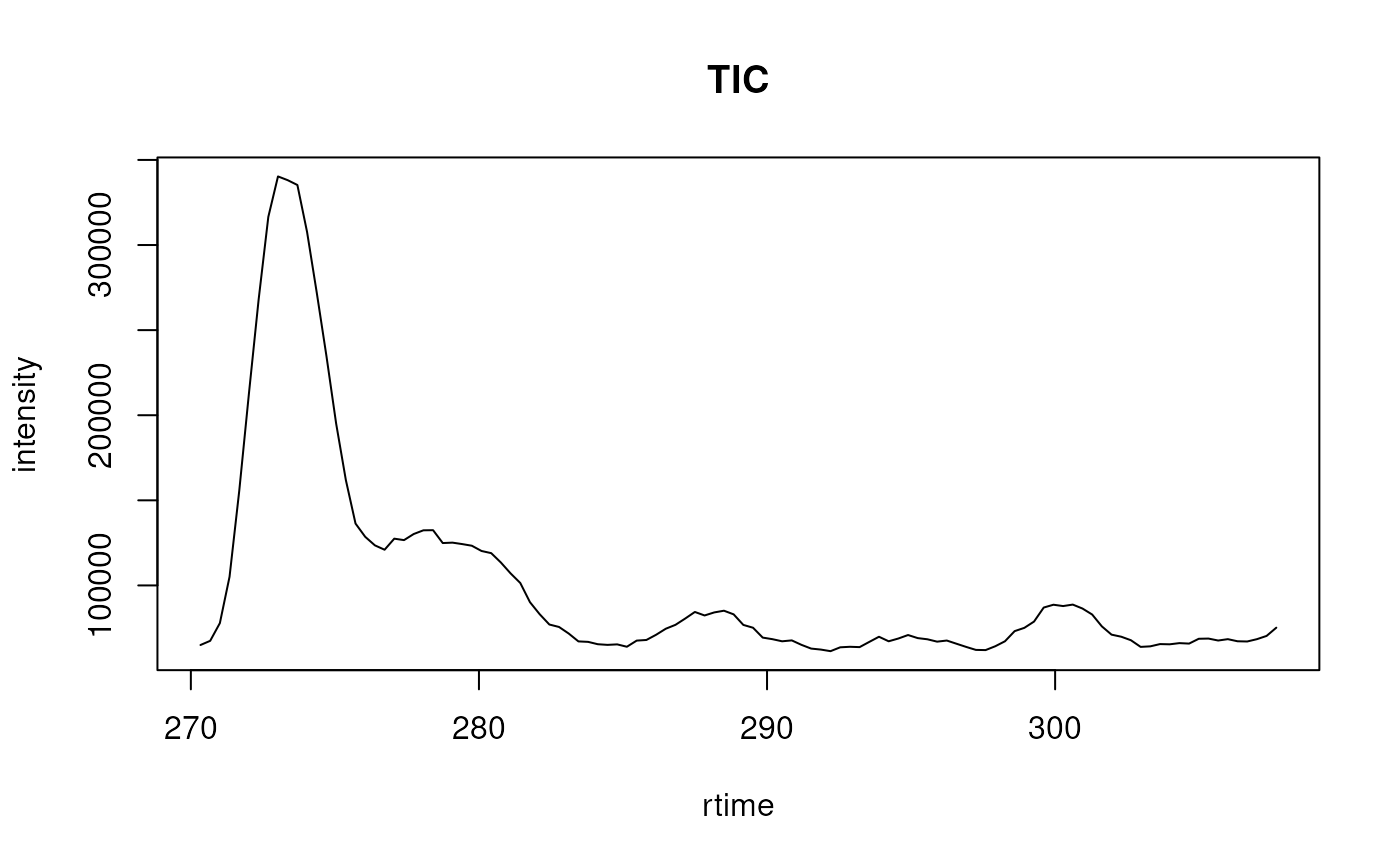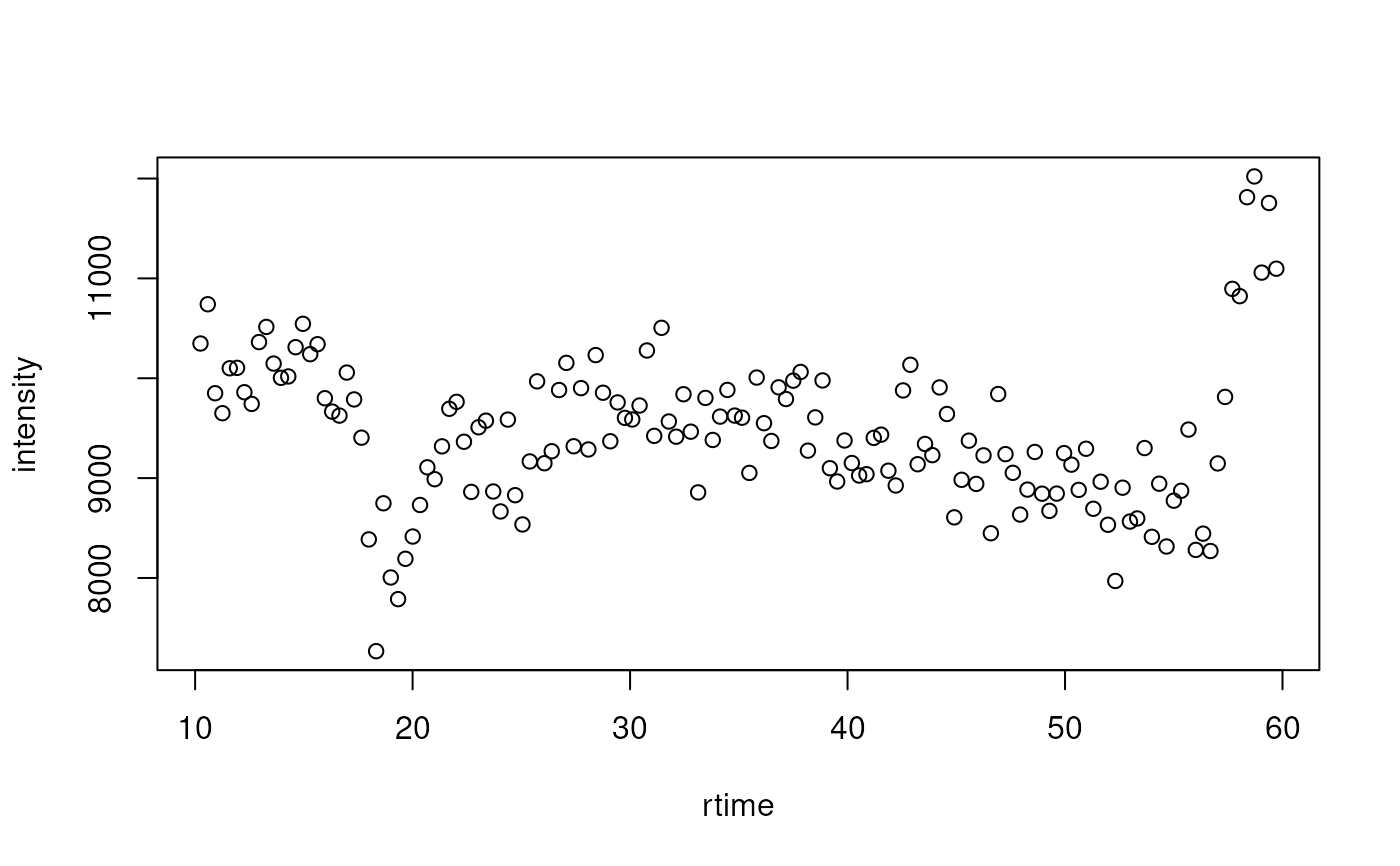The chromatogram method extracts chromatogram(s) from an MSnExp or OnDiskMSnExp object. Depending on the provided parameters this can be a total ion chromatogram (TIC), a base peak chromatogram (BPC) or an extracted ion chromatogram (XIC) extracted from each sample/file.

# S4 method for MSnExp
chromatogram(
object,
rt,
mz,
aggregationFun = "sum",
missing = NA_real_,
msLevel = 1L,
BPPARAM = bpparam()
)

Arguments

object For chromatogram: a MSnExp or OnDiskMSnExp object from which the chromatogram should be extracted. A numeric(2) or two-column matrix defining the lower and upper boundary for the retention time range/window(s) for the chromatogram(s). If a matrix is provided, a chromatogram is extracted for each row. If not specified, a chromatogram representing the full retention time range is extracted. See examples below for details. A numeric(2) or two-column matrix defining the mass-to-charge (mz) range(s) for the chromatogram(s). For each spectrum/retention time, all intensity values within this mz range are aggregated to result in the intensity value for the spectrum/retention time. If not specified, the full mz range is considered. See examples below for details. character defining the function to be used for intensity value aggregation along the mz dimension. Allowed values are "sum" (TIC), "max" (BPC), "min" and "mean". numeric(1) allowing to specify the intensity value for if for a given retention time (spectrum) no signal was measured within the mz range. Defaults to NA_real_. integer specifying the MS level from which the chromatogram should be extracted. Defaults to msLevel = 1L. Parallelisation backend to be used, which will depend on the architecture. Default is BiocParallel::bpparam().

Value

chromatogram returns a MChromatograms object with the number of columns corresponding to the number of files in object and number of rows the number of specified ranges (i.e. number of rows of matrices provided with arguments mz and/or rt). The featureData of the returned object contains columns "mzmin" and "mzmax" with the values from input argument mz (if used) and "rtmin" and "rtmax" if the input argument rt was used.

Details

Arguments rt and mz allow to specify the MS data slice from which the chromatogram should be extracted. The parameter aggregationSum allows to specify the function to be used to aggregate the intensities across the mz range for the same retention time. Setting aggregationFun = "sum" would e.g. allow to calculate the total ion chromatogram (TIC), aggregationFun = "max" the base peak chromatogram (BPC). The length of the extracted Chromatogram object, i.e. the number of available data points, corresponds to the number of scans/spectra measured in the specified retention time range. If in a specific scan (for a give retention time) no signal was measured in the specified mz range, a NA_real_ is reported as intensity for the retention time (see Notes for more information). This can be changed using the missing parameter.

By default or if \code{mz} and/or \code{rt} are numeric vectors, the
function extracts one \code{\link{Chromatogram}} object for each file
object. Providing a numeric matrix with argument \code{mz} or \code{rt}
enables to extract multiple chromatograms per file, one for each row in
the matrix. If the number of columns of \code{mz} or \code{rt} are not
equal to 2, \code{range} is called on each row of the matrix.

Chromatogram and MChromatograms for the classes that represent single and multiple chromatograms.

Johannes Rainer

Examples

## Read a test data file.

library(msdata)
f <- c(system.file("microtofq/MM14.mzML", package = "msdata"),
system.file("microtofq/MM8.mzML", package = "msdata"))

## Read the data as an MSnExp
msd <- readMSData(f, msLevel = 1)

## Extract the total ion chromatogram for each file:
tic <- chromatogram(msd)

tic
#> MChromatograms with 1 row and 2 columns
#>           MM14.mzML       MM8.mzML
#>      <Chromatogram> <Chromatogram>
#> [1,]    length: 112    length: 198
#> phenoData with 1 variables
#> featureData with 1 variables

## Extract the TIC for the second file:
tic[1, 2]
#> Object of class: Chromatogram
#> Intensity values aggregated using: sum
#> length of object: 198
#> from file: 2
#> mz range: [95.51765, 1005.043]
#> rt range: [0.486, 66.7818]
#> MS level: 1

## Plot the TIC for the first file
plot(rtime(tic[1, 1]), intensity(tic[1, 1]), type = "l",
xlab = "rtime", ylab = "intensity", main = "TIC")## Extract chromatograms for a MS data slices defined by retention time
## and mz ranges.
rtr <- rbind(c(10, 60), c(280, 300))
mzr <- rbind(c(140, 160), c(300, 320))
chrs <- chromatogram(msd, rt = rtr, mz = mzr)

## Each row of the returned MChromatograms object corresponds to one mz-rt
## range. The Chromatogram for the first range in the first file is empty,
## because the retention time range is outside of the file's rt range:
chrs[1, 1]
#> Object of class: Chromatogram
#> Intensity values aggregated using: sum
#> length of object: 0
#> from file: 1
#> mz range: [NA, NA]
#> MS level: 1

## The mz and/or rt ranges used are provided as featureData of the object
fData(chrs)
#>   mzmin mzmax rtmin rtmax polarity
#> 1   140   160    10    60        1
#> 2   300   320   280   300        1

## The mz method can be used to extract the m/z ranges directly
mz(chrs)
#>      mzmin mzmax
#> [1,]   140   160
#> [2,]   300   320

## Also the Chromatogram for the second range in the second file is empty
chrs[2, 2]
#> Object of class: Chromatogram
#> Intensity values aggregated using: sum
#> length of object: 0
#> from file: 2
#> mz range: [NA, NA]
#> MS level: 1

## Get the extracted chromatogram for the first range in the second file
chr <- chrs[1, 2]
chr
#> Object of class: Chromatogram
#> Intensity values aggregated using: sum
#> length of object: 148
#> from file: 2
#> mz range: [140.0022, 159.9989]
#> rt range: [10.24602, 59.71602]
#> MS level: 1

plot(rtime(chr), intensity(chr), xlab = "rtime", ylab = "intensity")